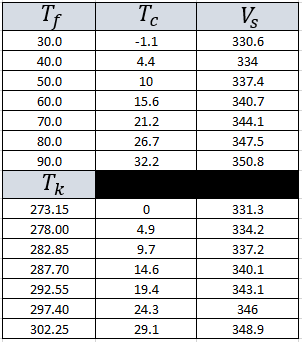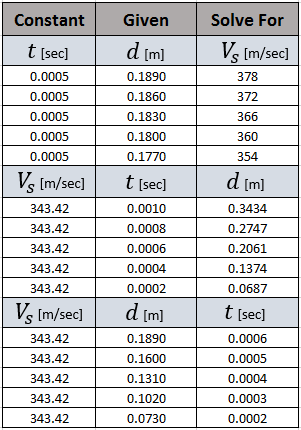# Skill Level 1

Quick Links to Main Topics:

Go To Bottom

Introduction to Skill Level 1
Speed of Sound
Distance Vs. Time

## Introduction to Skill Level 1

This section gives a quick overview of fundamental concepts used in this application. These concepts include: temperature and its effect on the speed of sound; and the relationship between distance, time, and speed/velocity. Skill level one is an introductory skill level and is meant for those with little or no exposure to the mathematics associated with sound and various types of waveforms.

In this module we will use the speed of sound and the time that it takes for the signal to reflect back to the emitting device to determine the distance of the object in question. How temperature effects the speed of sound and the calculations associated with determining the distance are discussed in further detail below.

## Speed of Sound

There are many factors that go into determining the exact speed of sound.The first thing to consider would be "what is the sound traveling through?" This may seem like an odd question but in fact, it matters. Sound "travels" differently depending on what type of material or "medium" it is traveling through. In short, whether it be a solid, liquid, or a gas. This is why things sound differently when you try to speak under water, or when you may have been trying to eavesdrop on a conversation through a wall. Using this application we know that the sound will be traveling through air.

Sound Traveling through Air:
When we are near the surface of the earth one of the greatest factors that effect the speed of sound is the temperature (We assume standard pressure and sea level). Many standard formulas for calculating the speed of sound use temperature in degrees centigrade, although it can be measured in many different ways. Below we have included conversions to degrees centigrade from both degrees Fahrenheit and Kelvin.

Temperature Conversions:

Let:

${T}_{c}=\text{Temperature in Degrees Celsius}\phantom{\rule{0ex}{0ex}}{T}_{f}=\text{Temperature in Degrees Fahrenheit}\phantom{\rule{0ex}{0ex}}{T}_{k}=\text{Temperature in Kelvin}$

From Degrees Fahrenheit to Degrees Centigrade:

${T}_{c}=\left({T}_{f}-32\right)x\left(\frac{5}{9}\right)$

Example: If the temperature outside is given to be 68° F what is the temperature in (°) Centigrade?

Given:${T}_{f}=68$° F

Solution:
$\phantom{\rule{0ex}{0ex}}\phantom{\rule{0ex}{0ex}}{T}_{c}=\left(68-32\right)x\left(\frac{5}{9}\right)\phantom{\rule{0ex}{0ex}}{T}_{c}=36x\left(\frac{5}{9}\right)\phantom{\rule{0ex}{0ex}}{T}_{c}=\left(\frac{36x5}{9}\right)\phantom{\rule{0ex}{0ex}}{T}_{c}=\left(\frac{180}{9}\right)\phantom{\rule{0ex}{0ex}}{T}_{c}=20°$

Therefore: We are able to say that 68° F is equivalent to 20° Centigrade.

From Kelvin to Degrees Celsius:

${T}_{c}={T}_{k}-273.15$

Example: If the temperature outside is given to be 300 K what is the temperature in (°) Centigrade?

Given:${T}_{k}=300$

Solution:
$\phantom{\rule{0ex}{0ex}}\phantom{\rule{0ex}{0ex}}{T}_{c}=300-273.15\phantom{\rule{0ex}{0ex}}{T}_{c}=26.85°$

Therefore: We are able to say that 300 Kelvin is equivalent to 26.85° C.(This is approximately 80° F)

Calculating the speed

Let:
${V}_{s}=\text{Speed of Sound}\phantom{\rule{0ex}{0ex}}{V}_{s}=331.3+\left(0.606x{T}_{c}\right)$

Example: If the temperature outside is given to be 20° C (Assume standard pressure and sea level), what is the approximate speed of sound.

Given: ${T}_{c}=20$° C

Solution:

${V}_{s}=331.3+\left(0.606x20\right)\phantom{\rule{0ex}{0ex}}{V}_{s}=331.3+\left(12.12\right)\phantom{\rule{0ex}{0ex}}{V}_{s}=343.42$

Therefore: We are able to say that at 20° C(68° F) the speed of sound is approximately 343.42 meters per second.

Knowing and being able to calculate the speed of sound is very useful in the next section. For this reason it is important that you understand the concepts above. Try some practice questions before moving on if you are unsure.

Practice:

The table below has been included so that you may practice. Test your knowledge by covering a column and attempting to calculate the others.## Distance and Time

There is a direct relationship between how fast you are moving, how long it takes, and the total distance traveled. This application uses this relationship to determine the distance to objects or if you choose the time it take to reach an object.

The speed of sound is treated as a constant because it is assumed there will not be a sudden change in temperature in the time that the application will be running(logical assumption). The general form of the speed of sound is:

Let:
${V}_{s}=\text{Speed of Sound}\phantom{\rule{0ex}{0ex}}d=\text{Distance to Object}\phantom{\rule{0ex}{0ex}}t=\text{Time Traveled}\phantom{\rule{0ex}{0ex}}{V}_{s}=\frac{d}{t}\phantom{\rule{0ex}{0ex}}\text{and has useful variations of:}\phantom{\rule{0ex}{0ex}}d=tx{V}_{s}\phantom{\rule{0ex}{0ex}}\text{or}\phantom{\rule{0ex}{0ex}}t=\frac{d}{{V}_{s}}$

Practice:

The table below has been included so that you may practice. Test your knowledge using the equations above and checking your answer.Note Displayed distances and times are to the object only. This application automatically computes the distance/time to object from the actual round trip measurements.

The signal actually travels to the object and back. This is known as round trip distance/time. If the object is stationary,then it can be assumed that the round trip distance is exactly twice the distance to the object. This can be represented by the following equation:

$2xd=tx{V}_{s}$